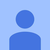+2

# Changing axis scale

Rob Maher 6 jaar geleden
Hi,

I am trying to use OxyPlot to show some data (*shock*).  My question is - how can the axis scale be changed once the data is plotted into it.

For example.  I use the following to create add the data to the graph

{
plotModel1.Title = "Data";
linearAxis1.TickStyle = OxyPlot.Axes.TickStyle.Inside;
linearAxis1.IsPanEnabled = true;
linearAxis1.IsZoomEnabled = true;

linearAxis2.Position = AxisPosition.Bottom;
linearAxis2.TickStyle = OxyPlot.Axes.TickStyle.Inside;
linearAxis2.IsPanEnabled = true;
linearAxis2.IsZoomEnabled = true;

plot1.Model = plotModel1;
}

and I want something like

public void Rescale()
{
linearAxis1.Minimum = 100;
linearAxis1.Maximum = 3200;

linearAxis2.Minimum = 200;
linearAxis2.Maximum = 2000;
plot1.Refresh();
plot1.InvalidatePlot(true);

plot1.Model.InvalidatePlot(true);
}

But this doesn't seem to do anything.  What am I missing here?  Any suggestions?

Thanks for looking.